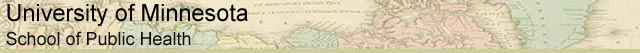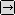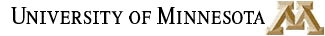### MATH REVIEW: USEFUL MATH FOR EVERYONE

SECTION 1. RATIOS AND PROPORTIONS

 Ratios and Proportions Algebraic Expressions Exponents Logarithms Glossary and Links

A ratio compares two numbers in order. Ratios are written with a colon, like this:

4:3

The order of numbers in a ratio is important. The ratio 4:3 expresses a different relationship than the ratio 3:4 does.

The units of measurement that each of the two numbers expresses is important too. The two numbers in a ratio do not have to have the same units. One chicken to every pot (1 chicken:1 pot) is a ratio. Saying that one British Pound is worth about 1.5 US dollars (1 GBP:1.5 USD) is another ratio.

In some cases, it can be convenient to express ratios all in the same units, for example, the ratio of 9 cups to 16 cups may be written in two ways: with a colon 9:16 or as a common fraction 9/16. The fraction 9/16 is another way of saying 9 divided by 16.

The two numbers in a fraction are referred to so often that they have been given names. The top number in a fraction is the numerator and the bottom number is the denominator:

numerator / denominator

A fraction is another way of expressing one number divided by another. What is 24/56? You can take out a calculator and enter

24 / 56 = 0.42857

Incidentally, if you wanted to know what percent this fraction represented, simply multiply the number by 100, or move the decimal two places to the right.to Ratios, Page 2